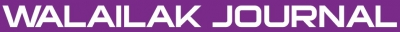### Finite Element Method: An Overview

Vishal JAGOTA, Aman Preet Singh SETHI, Khushmeet KUMAR

#### Abstract

The finite element method (FEM) is a numerical analysis technique for obtaining approximate solutions to a wide variety of engineering problems. A finite element model of a problem gives a piecewise approximation to the governing equations. The basic premise of the FEM is that a solution region can be analytically modeled or approximated by replacing it with an assemblage of discrete elements (discretization). Since these elements can be put together in a variety of ways, they can be used to represent exceedingly complex shapes.

#### Keywords

FEM, discretization, numerical analysis, approximate solution

PDF

#### References

RW Clough. The finite element method in plane stress analysis. In: Proceedings of the 2nd ASCE Conference on Electronic Computation, Pittsburgh, 1960.

R Courant. Variational methods for the solutions of problems of equilibrium and vibrations. Bull. Am. Math. Soc. 1943; 49, 1-23.

A Hrenikoff. Solution of problems in elasticity by the framework method. J. Appl. Mech. 1941; 8, 169-75.

D McHenry. A lattice analogy for the solution of plane stress problems. J. Inst. Civ. Eng. 1943; 21, 59-82.

NM Newmark. Numerical Methods of Analysis in Engineering. In: LE Grinter (ed.). Macmillan, New York, 1949.

G Kron. Tensorial analysis and equivalent circuits of elastic structures. J. Franklin Inst. 1944; 238, 399-442.

G Kron. Equivalent circuits of the elastic field. J. Appl. Mech. 1944; 66, A149-A161.

H Argyris. Energy theorems and structural analysis. Aircraft Eng. 1954; 26, 347-94.

H Argyris. Energy theorems and structural analysis. Aircraft Eng. 1955; 27, 42-158.

H Argyris. The matrix theory of statics. (in German) Ingenieur Archiv. 1957; 25, 174-92.

JH Argyris. The analysis of fuselages of arbitrary cross-section and taper. Aircraft Eng. 1959; 31, 62-283.

JH Argyris and S Kelsey. Energy theorems and structural analysis. Butterworth, London, 1960.

J Turner, RW Clough, HC Martin and LC Topp. Stiffness and deflection analysis of complex structures. J. Aeronaut. Sci. 1956; 23, 805-54.

C Zienkiewicz and YK Cheung. Finite elements in the solution of field problems. Engineer. 1965; 220, 507-10.

### Refbacks

• There are currently no refbacks.Online ISSN: 2228-835X

http://wjst.wu.ac.th

Last updated: 20 June 2019